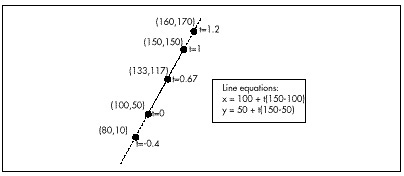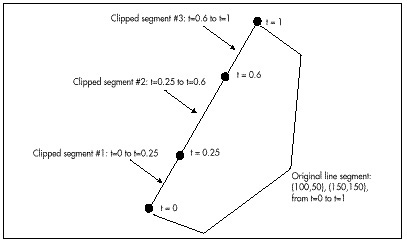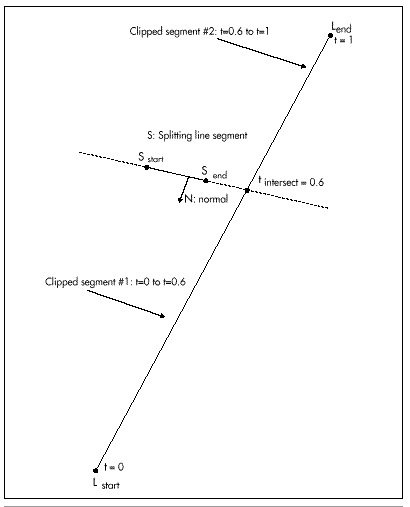If you find any of the above confusing (and it would be understandable if that were the case; BSP trees are not easy to get the hang of), you might want to refer back to the previous chapter. It would also be a good idea to get hold of the visual BSP compiler I’ll discuss shortly; when it comes to understanding BSP trees, there’s nothing quite like seeing one being built.

So there are really only two interesting operations in building a BSP tree: choosing a root node for the current subspace (a “splitter”) and assigning surfaces to one side or another of the current root node, splitting any that straddle the splitter. We’ll get to the issue of choosing splitters shortly, but first let’s look at the process of splitting and assigning. To do that, we need to understand parametric lines.

Parametric Lines

We’re all familiar with lines described in slope-intercept form, with y as a function of x

y = mx + b

but there’s another sort of line description that’s very useful for clipping (and for a variety of 3-D purposes, such as curved surfaces and texture mapping): parametric lines. In parametric lines, x and y are decoupled from one another, and are instead described as a function of the parameter t:

x = xstart + t(xend - xstart)
y = ystart + t(yend - ystart)

This can be summarized as

L = Lstart + t(Lend - Lstart)

where L = (x, y).

Figure 60.1 shows how a parametric line works. The t parameter describes how far along a line segment the current x and y coordinates are. Note that this description is valid not only for the line segment, but also for the entire infinite line; however, only points with t values between 0 and 1 are actually on the line segment.

In our 2-D BSP compiler (as you’ll recall from the previous chapter, we’re working with 2-D trees for simplicity, but the principles generalize to 3-D), we’ll represent our walls (all vertical) as line segments viewed from above. The segments will be stored in parametric form, with the endpoints of the original line segment and two t values describing the endpoints of the current (possibly clipped) segment providing a complete specification for each segment, as shown in Figure 60.2.

What does that do for us? For one thing, it keeps clipping errors from creeping in, because clipped line segments are always based on the original line segment, not derived from clipped versions. Also, it’s potentially a more compact format, because we need to store the endpoints only for the original line segments; for clipped line segments, we can just store pairs of t values, along with a pointer to the original line segment. The biggest win, however, is that it allows us to use parametric line clipping, a very clean form of clipping, indeed.Figure 60.1
A sample parametric line.Figure 60.2
Line segment storage in the BSP compiler.

Parametric Line Clipping

In order to assign a line segment to one subspace or the other of a splitter, we must somehow figure out whether the line segment straddles the splitter or falls on one side or the other. In order to determine that, we first plug the line segment and splitter into the following parametric line intersection equation

number = N (Lstart - Sstart) (Equation 1)
denom = -N (Lend - Lstart) (Equation 2)
tintersect = number / denom (Equation 3)

where N is the normal of the splitter, Sstart is the start point of the splitting line segment in standard (x,y) form, and Lstart and Lend are the endpoints of the line segment being split, again in (x,y) form. Figure 60.3 illustrates the intersection calculation. Due to lack of space, I’m just going to present this equation and its implications as fact, rather than deriving them; if you want to know more, there’s an excellent explanation on page 117 of Computer Graphics: Principles and Practice, by Foley and van Dam (Addison Wesley, ISBN 0-201-12110-7), a book that you should certainly have in your library.

If the denominator is zero, we know that the lines are parallel and don’t intersect, so we don’t divide, but rather check the sign of the numerator, which tells us which side of the splitter the line segment is on. Otherwise, we do the division, and the result is the t value for the intersection point, as shown in Figure 60.3. We then simply compare the t value to the t values of the endpoints of the line segment being split. If it’s between them, that’s where we split the line segment, otherwise, we can tell which side of the splitter the line segment is on by which side of the line segment’s t range it’s on. Simple comparisons do all the work, and there’s no need to do the work of generating actual x and y values. If you look closely at Listing 60.1, the core of the BSP compiler, you’ll see that the parametric clipping code itself is exceedingly short and simple.Figure 60.3
How line intersection is calculated.

One interesting point about Listing 60.1 is that it generates normals to splitting surfaces simply by exchanging the x and y lengths of the splitting line segment and negating the resultant y value, thereby rotating the line 90 degrees. In 3-D, it’s not that simple to come by a normal; you could calculate the normal as the cross-product of two of the polygon’s edges, or precalculate it when you build the world database.

The BSP Compiler

Listing 60.1 shows the core of a BSP compiler—the code that actually builds the BSP tree. (Note that Listing 60.1 is excerpted from a C++ .CPP file, but in fact what I show here is very close to straight C. It may even compile as a .C file, though I haven’t checked.) The compiler begins by setting up an empty tree, then passes that tree and the complete set of line segments from which a BSP tree is to be generated to SelectBSPTree(), which chooses a root node and calls BuildBSPTree() to add that node to the tree and generate child trees for each of the node’s two subspaces. BuildBSPTree() calls SelectBSPTree() recursively to select a root node for each of those child trees, and this continues until all lines have been assigned nodes. SelectBSP() uses parametric clipping to decide on the splitter, as described below, and BuildBSPTree() uses parametric clipping to decide which subspace of the splitter each line belongs in, and to split lines, if necessary.

Graphics Programming Black Book © 2001 Michael Abrash